Next: Identical Particles Up: Multi-Particle Systems Previous: Non-Interacting Particles

# Two-Particle Systems

Consider a system consisting of two particles, mass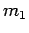and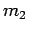, interacting via the potential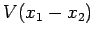which only depends on the relative positions of the particles. According to Eqs. (419) and (426), the Hamiltonian of the system is written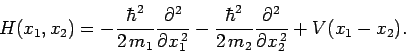(437)

Let(438)

be the particles' relative position, and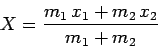(439)

the position of the center of mass. It is easily demonstrated that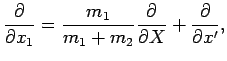(440)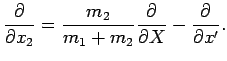(441)

Hence, when expressed in terms of the new variables,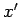and, the Hamiltonian becomes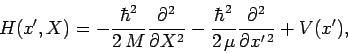(442)

where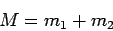(443)

is the total mass of the system, and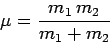(444)

the so-called reduced mass. Note that the total momentum of the system can be written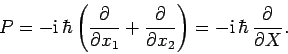(445)

The fact that the Hamiltonian (442) is separable when expressed in terms of the new coordinates [i.e.,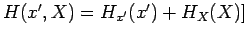suggests, by analogy with the analysis in the previous section, that the wavefunction can be factorized: i.e.,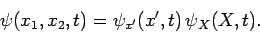(446)

Hence, the time-dependent Schrödinger equation (423) also factorizes to give(447)

and(448)

The above equation can be solved to give(449)

where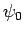,, and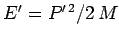are constants. It is clear, from Eqs. (445), (446), and (449), that the total momentum of the system takes the constant value: i.e., momentum is conserved.

Suppose that we work in the centre of mass frame of the system, which is characterized by. It follows that. In this case, we can write the wavefunction of the system in the form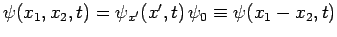, where(450)

In other words, in the center of mass frame, two particles of massand, moving in the potential, are equivalent to a single particle of mass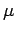, moving in the potential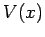, where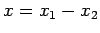. This is a familiar result from classical dynamics.Next: Identical Particles Up: Multi-Particle Systems Previous: Non-Interacting Particles
Richard Fitzpatrick 2010-07-20